# Writing a simple Compiler on my own - Action Rules for Function Declarations (part 2) [C][Flex][Bison]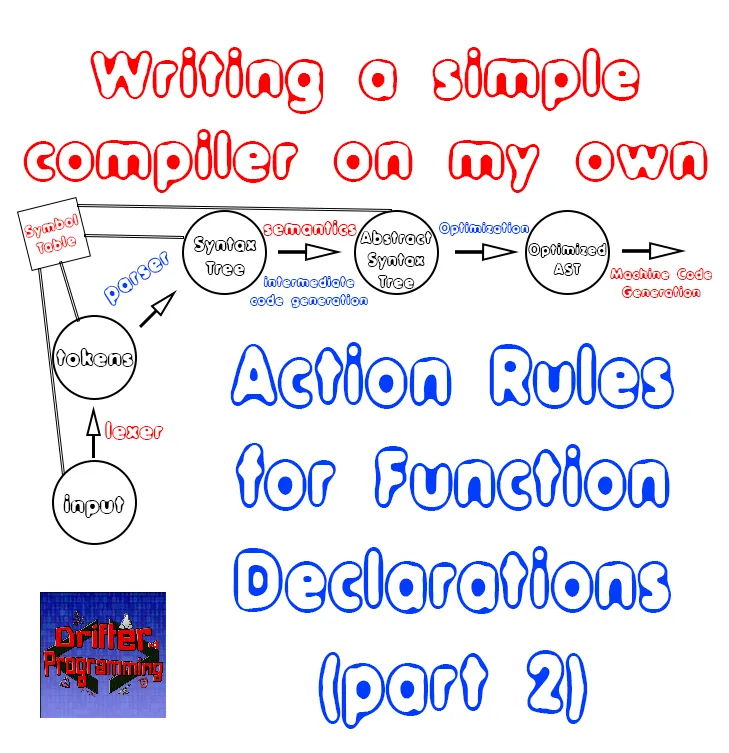[Custom Thumbnail]

All the Code of the series can be found at the Github repository:
https://github.com/drifter1/compiler

# Introduction

Hello it's a me again @drifter1! Today we continue with my Compiler Series, a series where we implement a complete compiler for a simple C-like language by using the C-tools Flex and Bison. This is part 2 of the previous article that you should go and read before-hand! Today we will actually use all the AST Nodes and functions that we wrote last time as action code in our parser!

## More specifically, the topics that we will cover today are:

1. Action Rules for Function declarations
2. Action Rules for Function declaration
3. Action Rules for Parameters
4. Traversing after parsing is done using "program"
5. Compiler validation using examples

## Requirements:

Actually you need to read and understand all the topics that I covered in the series as a whole, as these articles will give you access to knowledge about:
• What Compiler Design is (mainly the steps)
• For which exact Language the Compiler is build for (Tokens and Grammar)
• How to use Flex and Bison
• How to implement a lexer and parser for the language using those tools
• What the Symbol Table is and how we implement it
• How we combine Flex and Bison together
• How we can pass information from the Lexer to the Parser
• How we define operator priorities, precedencies and associativity
• What Semantic Analysis is (Attributes, SDT etc.)
• How we do the so called "Scope Resolution"
• How we declare types and check the type inter-compatibility for different cases, including function parameters
• How we check function calls later on using a "Revisit queue", as function declarations mostly happen after functions get used (in our Language)
• Intermediate Code Representations, including AST's
• How we implement an AST (structure and management)
• Action Rules for other cases

## Difficulty:

Talking about the series in general this series can be rated:
Today's topic(s) can be rated:
• Medium

# Actual Tutorial Content

## Action Rules for Function declarations

Continuing on with function declarations now, we know that functions are optional. So, we first have to pass the information from the actual "functions" rule that has to store a function declarations node to the "functions_optional" rule. When there's no function declaration we will pass NULL.

We can visualize this as following (from last time):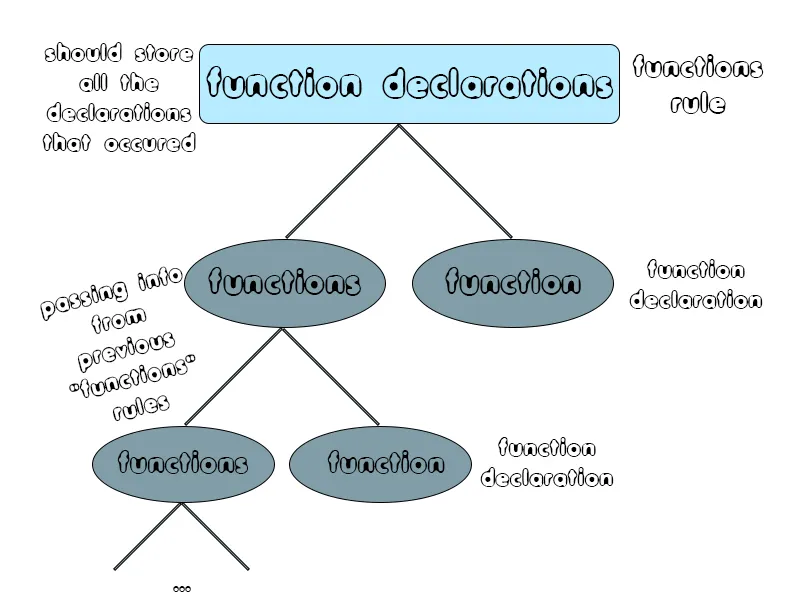So, the "functions_optional"-rule looks like this:

``````functions_optional:
functions
{
\$\$ = \$1;
}
| /* empty */
{
\$\$ = NULL;
}
;``````

For the actual "functions" rule that gives us a Function Declarations node we will have to use the node creation function. The parameters will be gotten from the declarations array and declaration count of a previous "function" non-terminal or will be NULL and 0, when it's the first declaration. The function declaration that's to be added will be given as the third and final parameter! So, the "functions"-rule looks as following:

``````functions:
functions function
{
AST_Node_Func_Declarations *temp = (AST_Node_Func_Declarations*) \$1;
\$\$ = new_func_declarations_node(temp->func_declarations, temp->func_declaration_count, \$2);
}
| function
{
\$\$ = new_func_declarations_node(NULL, 0, \$1);
}
;``````

But, to do this we also have to set up the function declaration...

## Action Rules for Function declaration

As we already said last time, we have to create a global temporary variable that stores the current function declaration node. That way the "far" childs of the "function"-rule will be able to access and change the information from this node. So, the parser now starts with:
``````%{
...
// for functions
AST_Node_Func_Decl *temp_function;
%}``````

The functions-rule already has some actions that manage the scope. We increase it before the function_head hide it after the function_tail, and so function declaration is over. Knowing that the function node will be stored in the "temp_function" variable, we just have to type-cast this node and give it directly to the function rule! So, the action code of "functions" is:
``````function: { incr_scope(); } function_head function_tail
{
hide_scope();
\$\$ = (AST_Node *) temp_function;
}
;``````

The visualization of a function declaration, that I also had last time is: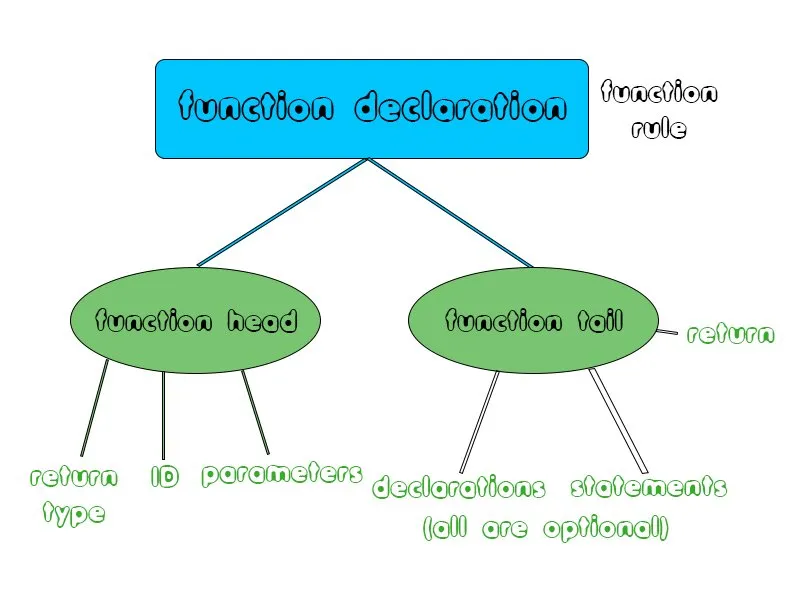Things start getting interesting in the function_head rule, where we also manage if we are declaring using the "declare" variable (if you remember). After "finding" the return type, pointer "flag" and symbol table entry, which are stored inside of the return_type node and ID itself, we can create the temporary function node! After type-casting the Return Type node we can access the ret_type and pointer variable, which can be easily passed to the node creation function, which will return the node to "temp_function". After doing that we should also set the type of the symbol table entry to "FUNCTION_TYPE" and the pointing type stored in "inf_type" to the return type of the function, that can be gotten from many points. After that the rule continues, managing the parameters, that I will leave for later...
So, let's get into the "current" action code of function_head:
``````function_head: { declare = 1; } return_type ID LPAREN
{
declare = 0;
AST_Node_Ret_Type *temp = (AST_Node_Ret_Type *) \$2;
temp_function = (AST_Node_Func_Decl *)
new_ast_func_decl_node(temp->ret_type, temp->pointer, \$3);
temp_function->entry->st_type = FUNCTION_TYPE;
temp_function->entry->inf_type = temp->ret_type;
}
...
;``````

### Return Type

The return type is pretty easy to set up as we already know the type that is stored in "type" (in form of an integer) and only have to give '0' or '1' as second parameter of the node creation function, showing if we have a pointer or not. So, the "return_type"-rule looks as following:
``````return_type:
type
{
\$\$ = new_ast_ret_type_node(\$1, 0);
}
| type pointer
{
\$\$ = new_ast_ret_type_node(\$1, 1);
}
;``````

### Optionals

The optional declarations, statements and return node are also pretty simple. In the first two we just have to set the entry of the temporary function node "temp_function" to the declarations or statements node, or NULL if there's no declaration or statement. In the same way the entry "return_node" of the temporary node will be set to NULL or to the result that we get from the node creation function. For now, where we don't know the data type of expressions, we will just expect the return expression to have the same type as the temporary function node. So, these three function_tail sub-rules look as following:
``````declarations_optional:
declarations
{
temp_function->declarations = \$1;
}
| /* empty */
{
temp_function->declarations = NULL;
}
;
statements_optional:
statements
{
temp_function->statements = \$1;
}
| /* empty */
{
temp_function->statements = NULL;
}
;
return_optional:
RETURN expression SEMI
{
temp_function->return_node = new_ast_return_node(temp_function->ret_type, \$2);
}
| /* empty */
{
temp_function->return_node = NULL;
}
;``````

Of course all these, including function_tail, don't store any information! These nodes are of no actual type! We could of course get into a different implementation, but this way is much simpler to understand :)

## Action Rules for Parameters

Parameters can be visualized as following (from last time):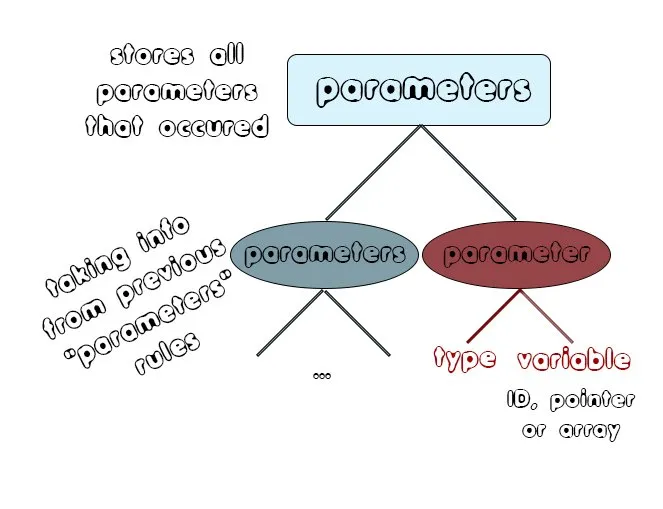Parameters are also optional and so the "parameters_optional" has to get it's value from the "parameters" rule. When there's no parameter we will pass NULL. So, this rule looks as following:
``````parameters_optional:
parameters
{
\$\$ = \$1;
}
| /* empty */
{
\$\$ = NULL;
}
;``````

The parameters-rule will use the node creation function, similar to the function declarations. This means that we will be adding the newly found parameter to the parameters array that we get from the previous parameter declarations node, by also incrementing the counter of parameters. When the parameter is the first or only parameter then we will pass NULL and 0 as parameters of the node creation function, else we will pass the "previous" array and counter, that has been gotten from the previous node. So, the "parameters"-rule looks as following:
``````parameters:
parameters COMMA parameter
{
AST_Node_Decl_Params *temp = (AST_Node_Decl_Params *) \$1;
\$\$ = new_ast_decl_params_node(temp->parameters, temp->lnum_of_pars, \$3);
}
| parameter
{
\$\$ = new_ast_decl_params_node(NULL, 0, \$1);
}
;``````

Defining the parameter non-terminal as a Param structure and knowing that we have defined a function called "def_param" that allows the creation of parameters with a specific data type, name and "reference way" (which is not needed here), we can easily set up this new parameter such as:
``````parameter : { declare = 1; } type variable
{
declare = 0;
\$\$ = def_param(\$2, \$3->st_name, 0);
}
;``````

Of course we also set the value of "declare" correspondingly to '1' and '0' afterwards, as we are declaring new variables!

After making the parameters we can now finish the function_head rule. The parameters and number of parameters will be stored in the symbol table entry of the function that we are able to function though the temporary function node. When there's no parameter we will pass NULL and 0, else we will type-cast the parameters_optional node to access the array and counter of it. So, in the end the "function_head"-rule becomes:
``````function_head: { declare = 1; } return_type ID LPAREN
{
declare = 0;
AST_Node_Ret_Type *temp = (AST_Node_Ret_Type *) \$2;
temp_function = (AST_Node_Func_Decl *)
new_ast_func_decl_node(temp->ret_type, temp->pointer, \$3);
temp_function->entry->st_type = FUNCTION_TYPE;
temp_function->entry->inf_type = temp->ret_type;
}
parameters_optional RPAREN
{
if(\$6 != NULL){
AST_Node_Decl_Params *temp = (AST_Node_Decl_Params *) \$6;
temp_function->entry->parameters = temp->parameters;
temp_function->entry->num_of_pars = temp->num_of_pars;
}
else{
temp_function->entry->parameters = NULL;
temp_function->entry->num_of_pars = 0;
}
}
;``````

## Traversing after parsing is done using "program"

Instead of printing each node on it's own, causing duplicate nodes and a difficulty understanding what the compiler "found", we will traverse the declarations, statements and function_optional non-terminals. The first stores the declarations in form of a declarations nodes. The second stores the statements in form of a statements node. The last one stores the function declarations inside of a function declaration nodes. So, traversing each one of them, we can easily traverse the whole program!

So, the new program-rule looks as following:
``````
program:
declarations { ast_traversal(\$1); }
statements   { ast_traversal(\$3); }
RETURN SEMI functions_optional { ast_traversal(\$7); }
;``````

## Compiler valdation using examples

After doing that we can now get into some examples. Let's split them up based on the number of functions.

### Example.c with no functions

The first example contains no functions at all. The code of it looks as following:
``````int i;
double val, res;
val = 2.5;
res = val + 1;
return;``````

Let's run the compiler for it to see what we get: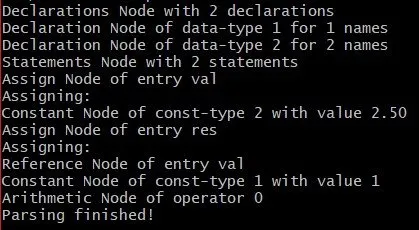As you can see the compiler worked perfectly without functions, as we wanted!

### Example2.c with one function

The code of this example is:
``````int i;
double val = 2.5, res;
for(i = 0; i < 10; i++){
res[i] = operation(val, i);
val = res[i];
print(res[i]);
print('\n');
}
return;
double operation (double value, int i){
// declarations
double res;
// statements
res = value*i + i;
return res;
}``````

Running the compiler for this example we get: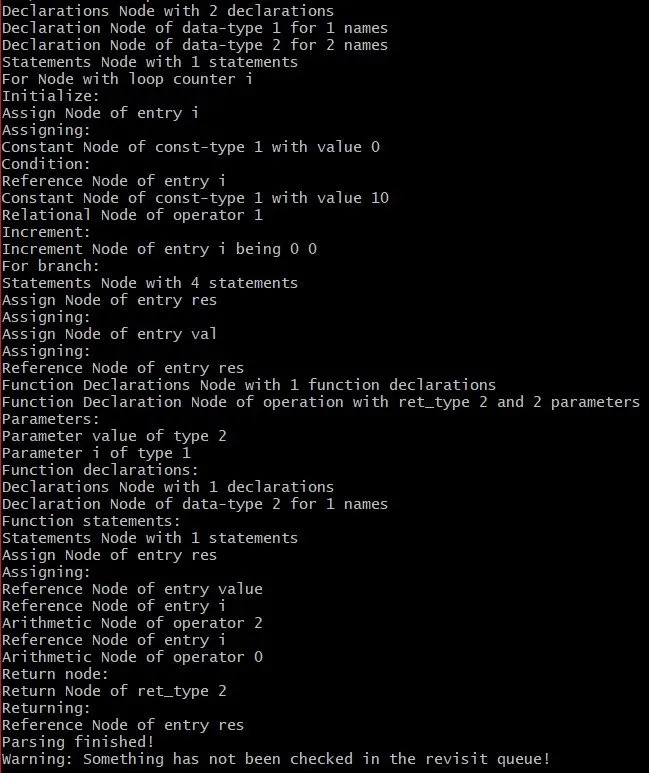You can see that the compiler found:
• the data type double which is represented by '2'
• the function name "operation"
• The two parameters "value" and 'i', which are of type '2' and '1' that represent double and integer respectively
• The declaration of the double "res"
• The assignment statement of "res", and with the correct post-fix order of operators
• The return node that returns the identifier "res"

### Full_example.c with 3 functions

Let's finally also run it for the "famous" example that contains most of the statements of the grammar. The code of it is:
``````// declarations
int i;                    // simple variable
char c = 'c';             // one with init
double val = 2.5, res; // two variables, one with init and one array
double *p;                // pointer variable
// statements
p = &res;
for(i = 0; i < 10; i++){
if(i > 5){
break;
}
else if(i == 5){
i = 2 * i;
val = func1();
print(res[i]);
print("\n");
continue;
}
else{
val = res[i];
print(res[i]);
print("\n");
p = p + 1;
}
if(i == 2 && val == 4.5){
print("iteration: 3\n");
}
}
while(i <<12){
print(i);
print(" ");
func2(c);
i++;
}
print("\n");
return; /* RETURN SEMI */
// other functions (functions)
int func1(){        /* without parameters */
return 5;
}
void func2(char c){ /* with one parameter */
char *s;
*s = c;
print(*s);
}
double add (double a, int b){  /* with two parameters */
double res;
res = a + b + (-5);
return res;
}``````

Running the compiler for it we get: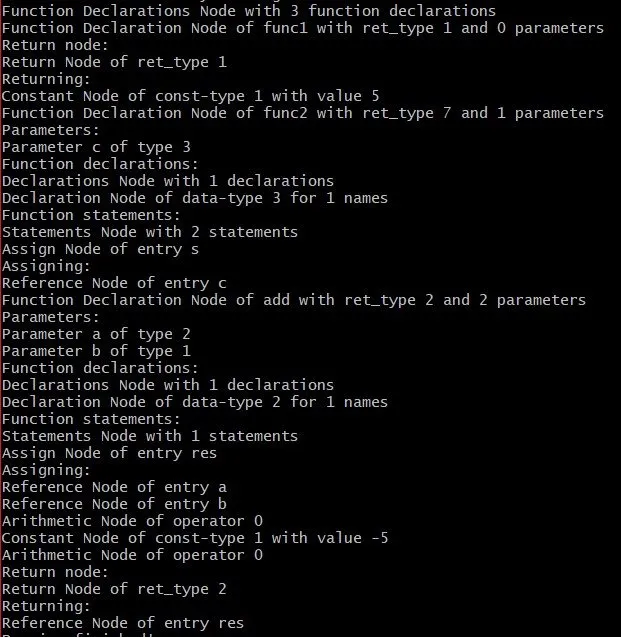The compiler truly found that:
• we have 3 functions
• the first function has no parameters, declarations and statements, but only a return node of '5'
• the second function has one parameter (char 'c' represented by data type '1'), one declaration (of one variable/name with data type '2', which is double) and two statements (an assignment of 's' and the "invisible for now" print function call)
• the third function which has two parameters, one declaration, a somewhat complicated statement and a return node

## RESOURCES

### References:

No references, just using code that I implemented in my previous articles.

### Images:

All of the images are custom-made!

## Final words | Next up on the project

And this is actually it for today's post! I hope that I explained everything as much as I needed to, meaning that you learned something out of it.
Next up on this series are:
• Semantic analysis (using more action rules in Bison)
• Intermediate Code generation (using the AST structure for more cases)
• Machine Code generation (MIPS Assembly)
Which are all topics that will need more than one article to complete. Also, note that we might also get into Optimizations later on, or could even extend the Language by adding complex datatypes (structs and unions), more rules etc.
So, see ya next time!

## GitHub Account:

https://github.com/drifter1Keep on drifting! ;)

H2
H3
H4
3 columns
2 columns
1 column